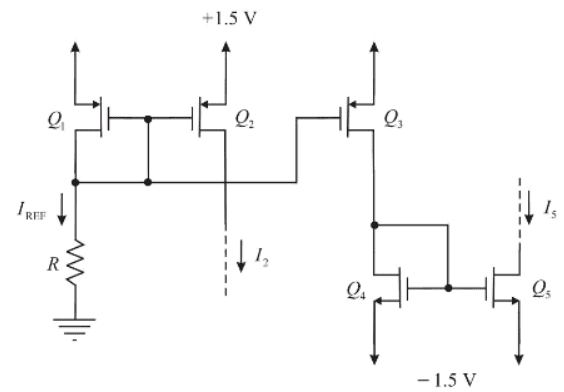# Simple Integrated Circuit Question

• Engineering
I have a simple conceptual question rather than an actual problem so pardon me for not using the provided template.

There's a question in my Microelectronic Circuits book involving this circuit:where it states that
voltages at the drain of Q2 can be as high as +1.3 V and voltages at the drain of Q5 can be as low as -1.3 V.

Part of the solution involves this equation:

VOV4 = VD4 - VS4 (equation for overdrive voltage of Q4)

VOV4 = -1.5 - (-1.3)

My question is: Why is VD4 equal to -1.5 V and why is VS4 equal to -1.3 V? -1.3 V is the voltage at the drain of Q5, how did it become the voltage at the source of Q4?

rude man
Homework Helper
Gold Member
My question is: Why is VD4 equal to -1.5 V and why is VS4 equal to -1.3 V? -1.3 V is the voltage at the drain of Q5, how did it become the voltage at the source of Q4?
Unfortunately, what you state is incorrect. The drain of D4 will be more positive than the source.
The drain of Q4 is > -1.5V. Perhaps as low as -1.3V but certainly no lower.
The source of Q4 is at -1.5V.
The drain of Q5 can be as low as -1.3V.
For an NMOS. the drain is more positive than the source unless it's operated in the "inverse" mode in which case the labels "drain" and "source" become a matter of semantics. I have never encountered a circuit using the inverse mode, except once which turned out to be a design error. So forget the inverse mode.

Unfortunately, what you state is incorrect. The drain of D4 will be more positive than the source.
The drain of Q4 is > -1.5V. Perhaps as low as -1.3V but certainly no lower.
The source of Q4 is at -1.5V.
The drain of Q5 can be as low as -1.3V.
For an NMOS. the drain is more positive than the source unless it's operated in the "inverse" mode in which case the labels "drain" and "source" become a matter of semantics. I have never encountered a circuit using the inverse mode, except once which turned out to be a design error. So forget the inverse mode.

Turns out the solution manual had it wrong... it should be VOV4 = -1.3 - (-1.5)

New question concerning same circuit: Why is R = (VS2 + VGS2)/IREF? Shouldn't it be VD1/IREF?

NascentOxygen
Staff Emeritus
Both seem correct.

donpacino
Gold Member
New question concerning same circuit: Why is R = (VS2 + VGS2)/IREF? Shouldn't it be VD1/IREF?

VGS2=VG2-VS2
VS2+VGS2=VG2

VD1=VG2

due to symmetry VD1=VD2
therefore R=(VS2+VGS2)/Iref=VD1/IREF=VD2/IREF

New question related to same circuit in the initial post: the solution manual states that VDS2max = VOV2 = VGS2 - Vth. My question is why is VDS2max = VOV2? Shouldn't VDS2 = VGS2 since the drain and gate of Q2 are connected?

rude man
Homework Helper
Gold Member
New question related to same circuit in the initial post: the solution manual states that VDS2max = VOV2 = VGS2 - Vth. My question is why is VDS2max = VOV2? Shouldn't VDS2 = VGS2 since the drain and gate of Q2 are connected?
VDS2max is the max allowed D-S voltage. Yes, obviously, VDS2 = VGS2 so therefore you can also say that VOV2 = VGS2max.

Isn't VOV = VGS - Vth, so shouldn't VOV2 = VGS2max - Vth? (or VOV2 = VDS2max - Vth) rather than VOV2 = VGS2max?

rude man
Homework Helper
Gold Member
Isn't VOV = VGS - Vth, so shouldn't VOV2 = VGS2max - Vth? (or VOV2 = VDS2max - Vth) rather than VOV2 = VGS2max?
VOV in general just means "overvoltage". It could apply to any two transistor terminals.

VOV in general just means "overvoltage". It could apply to any two transistor terminals.
I get that but what about the threshold voltage (Vth)? The overvoltage is the voltage across two transistor terminals minus the threshold voltage. Why don't we include it in the equation for VOV = VDS2max?

rude man
Homework Helper
Gold Member
I get that but what about the threshold voltage (Vth)? The overvoltage is the voltage across two transistor terminals minus the threshold voltage.
No. The overvoltage is the maximum voltage you can apply between any two terminals. It has nothing to do with Vth or any other transistor parameter.

No. The overvoltage is the maximum voltage you can apply between any two terminals. It has nothing to do with Vth or any other transistor parameter.

Ah ok. I was confusing it with the overdrive voltage; I thought they were the same thing.